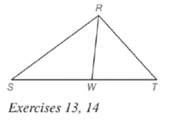Chapter 5.6, Problem 13EElementary Geometry For College St...

7th Edition
Alexander + 2 others
ISBN: 9781337614085

Solutions

Chapter
SectionElementary Geometry For College St...

7th Edition
Alexander + 2 others
ISBN: 9781337614085
Textbook Problem

Given: R W → bisects ∠ S R T Do the following equalities hold? a) S W = W T b) R S R T = S W W TTo determine

a)

To check: Whether the equality SW=WT holds or not.

Explanation

Given:

RW bisects SRT.

Theorem used:

Angle bisector theorem:

If a ray bisects one angle of a triangle, then it divides the opposite side into segments whose lengths are proportional to the lengths of the two sides that form the bisected angle

To determine

b)

To check: Whether the equality RSRT=SWWT holds or not.

Still sussing out bartleby?

Check out a sample textbook solution.

See a sample solution

The Solution to Your Study Problems

Bartleby provides explanations to thousands of textbook problems written by our experts, many with advanced degrees!

Get Started

In Problems 15-28, perform the operations if possible. 24. .

Mathematical Applications for the Management, Life, and Social Sciences

Simplify the expressions in Exercises 97106. 21/32122/321/3

Finite Mathematics and Applied Calculus (MindTap Course List)

In Exercises 107-120, factor each expression completely. 120. x3 27

Applied Calculus for the Managerial, Life, and Social Sciences: A Brief Approach

Solve each inequality. x2

Trigonometry (MindTap Course List)

The distance from (1, 2, 1) to the plane 6x + 5y + 8z = 34 is:

Study Guide for Stewart's Multivariable Calculus, 8th

True or False: f(x)f(a)xa may be interpreted as the instantaneous velocity of a particle at time a.

Study Guide for Stewart's Single Variable Calculus: Early Transcendentals, 8th

Solve each equation. x42x2+1=0

College Algebra (MindTap Course List)

Proof Prove that cotudu=lnsinu+C.

Calculus (MindTap Course List)